HomePuzzle ContestSingle Shift PuzzleFlip Flat RubikDouble Shift PuzzleRotate 3x3 PuzzleRotate 5x5 PuzzleRotate 7x7 PuzzleNine Faces RubikSix Faces Rubik24 Faces Magic Cube4D Rubik CubeQuotes By AuthorQuotes By SubjectQuote Of The DayDetective MysteryReversiLogic TestMath TestWord Of The DayUnsolved ProblemsHall of FameContact UsLoginSign UpSign OutMy AccountChange PasswordAbout UsContributorsLink To UsPrivacy PolicyTerms of ServiceSite Map

## Six Faces Flat Rubik Puzzle

Six Faces Flat Rubik puzzle is a two-dimensional equivalent of the original 3D Rubik's Cube. Six Faces Flat Rubik Puzzle is a variation of Nine Faces Flat Rubik Puzzle with an additional movement restriction on three out of nine colored blocks. Six Faces Flat Rubik 2D puzzle is an equivalent to the original 3D Rubik's Cube puzzle. For the puzzle to be solved, colors should be separated and each block must be a solid color (see the left image in Figure 1).
You can rotate 3x3 square block and shift 12 elements presenting one of the square's angles on each move. There are two options: Rotate square and shift angle Clockwise and Rotate square and shift angle Counterclockwise. There are 24 square marked with white numbers. By clicking on the white number square, you can rotate a 3x3 square block 90 degrees and shift 12 elements presenting the closest square's angle.
For example, if you select the 'Rotate and Shift Clockwise' option and click on “39” square, 3x3 square block 37-39-45-43 will rotate clockwise 90 degrees (see Figure 1). In addition it will shift Clockwise 12 elements presenting the closest square's angle (7,8,9=>16,17,18=>46,49,52=>73,76,79=>7,8,9).Figure 1
Columns are enumerated from #1 to #9. Next column after column #9 we define as a column #1 again. The previous column for column # 1 is column #9. The same rule applies to the rows.Figure 2
For example, if you select the 'Rotate and Shift Clockwise' option and click on #3 square (white "X" square next to #2 square), 3x3 square block 1-3-9-7 will rotate clockwise 90 degrees (see Figure 2). In addition it will shift clockwise 12 elements presenting the closest square's angle (79,80,81=>61,62,63=>10,13,16=>37,40,43=>79,80,81).
6 Faces Flat Rubik Puzzle allows 6 clockwise rotations and 6 counterclockwise rotations.
Rotation Green face clockwise (see Figure 3).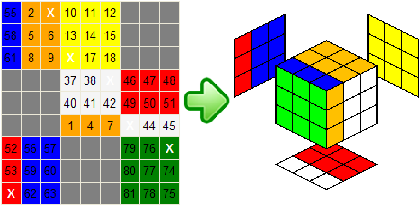Figure 3
Rotation Blue face clockwise (see Figure 4).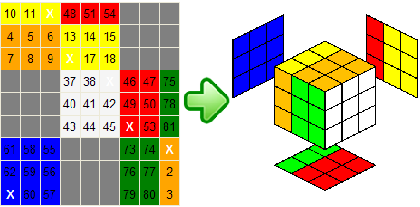Figure 4
Rotation Red face clockwise (see Figure 5).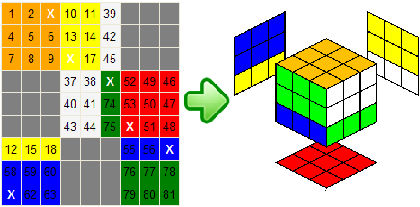Figure 5
Rotation Orange face clockwise (see Figure 6).Figure 6
Rotation White face clockwise (see Figure 7).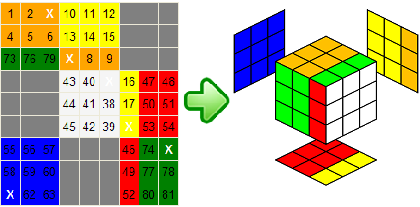Figure 7
Rotation Yeelow face clockwise (see Figure 8).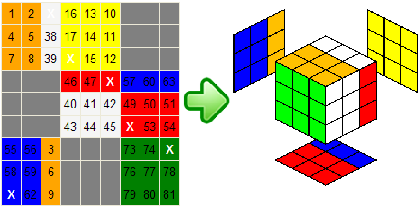Figure 8